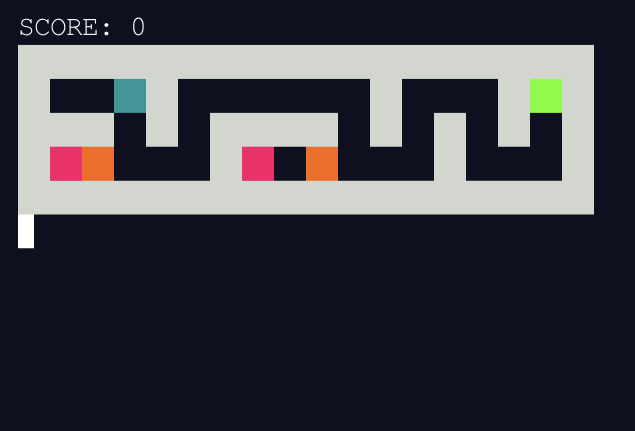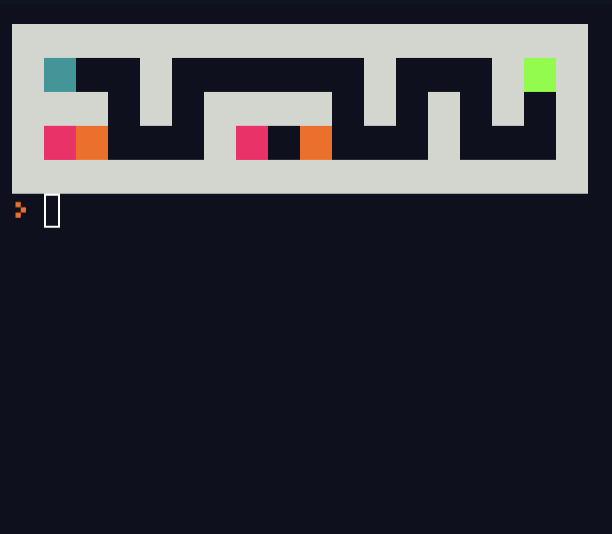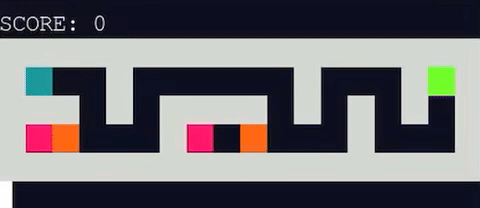Learn to Code via Tutorials on Repl.it!

← Back to all posts

# Make Your Own Maze Game in Python Shell!

Hi! This is a tutorial on how to make a maze game (and more!) in Python! Some functions are from my previous project: Blue Square Adventures### 1: Get Ready

First of all, let’s import some modules.

``````from getch import getch
from os import system``````

Getch allows us to detect single key presses, while `os.system(“clear”)` will clear the screen for each frame in our maze game.

Now, we want to perhaps have prizes in the maze you could collect. They’ll give you some points so we will need to assign 0 to the variable score.

``score = 0``

Design a maze level for the game. Then, put it in a list called game and create another one called original_game which is the game without the character. You can design more complex levels, but for now, I’ll just use this one. “C” will be the player or character, “D” will stand for danger, “\$” gives you points, and “F” is the finish.

``````maze_level=Your level here as multi line string
game=maze_level.splitlines()
original_game=maze_level.replace("C"," ").splitlines()``````

Also, we will need a `replace_string()` function to replace a character of a string from a certain index.

``````def replace_string(text,index,substring):
new = list(text) #Turns text into list
new[index] = substring #Changes the character
return ''.join(new) #Turns it back into a string and return``````

We’ll need this function later.

### 2: Fruitful Functions

Some of these functions prove to be extremely useful when making games in Python.
`row()` and `col()` will basically get the coordinates of our character by searching in the list. In here, let’s say that “C” is the character of the game.

``````def row():
for i in range(len(game)):
if "C" in game[i]:
return i
def col():
global game
for i in game:
for j in range(len(i)):
if "C" == i[j]:
return j``````

These functions will be used in our next functions, as well as later in the code.

The functions that move our character are the most important.

``````def up():
c=col()
r=row()
square=game[row()][col()] #Makes function flexible whatever character you use
game[r]=original_game[r] #Makes player disappear
game[r-1]=replace_string(game[r-1],c,square)#Makes it reappear at a different position

def down():
c=col()
r=row()
square=game[row()][col()]
game[r]=original_game[r]
game[r+1]=replace_string(game[r+1],c,square)

def left():
c=col()
r=row()
square=game[row()][col()]
game[r]=replace_string(game[r],c,original_game[r][c])
game[r]=replace_string(game[r],c-1,square)

def right():
c=col()
r=row()
square=game[row()][col()]
game[r]=replace_string(game[r],c,original_game[r][c])
game[r]=replace_string(game[r],c+1,square)``````

These functions move the player up, down, left, or right. They replace the row the player is on with the same row from original_game so the player disappears. Then, it places the player on its new position.
We’d also like our game to look colorful.

``````def color(string):
return string.replace(" ","  ").replace("#","\u001b[47;1m  \u001b[0m").replace("F","\u001b[42;1m  \u001b[0m").replace("C","\u001b[46;1m  \u001b[0m").replace("D","\u001b[41m  \u001b[0m").replace("\$","\u001b[43;1m  \u001b[0m")``````

This function here replaces text characters in the game with colorful squares (using background color ansi codes)
Let’s test this function out! Add the line `for i in game: print(color(i))` to the code temporarily. It should look like this when it runs.### 3: The Main Loop (a.k.a. good stuff)

Of coarse we need to make the game playable. Here we can add a loop for it. The loop will take inputs (with getch) and print the game.

``````while True:
#Clear Screen
system("clear")
#Print Game
print("SCORE: "+str(score))
for i in game:
print(color(i))

#Take input
try:
ans = getch()
except:
ans = ''``````

Make sure to use `try:` around `getch()`. Sometimes it shows a weird overflow error if I leave it there for too long.

Now that we have the variable `ans`, which is our input, let’s start processing it. First, we need to make the movement.

``````if ans == "w" and game[row()-1][col()] != "#":
up()
elif ans == "s" and game[row()+1][col()] != "#":
down()
elif ans == "a" and game[row()][col()-1] != "#":
left()
elif ans == "d" and game[row()][col()+1] != "#":
right()``````
``Put this in the loop.``

The purpose of `game[row()-1][col()] != "#"` is to make sure that there is no wall in front of the player. If this didn’t exist, the player will be able to walk through walls.

The next step is to check the player’s position.

``````# Has the player gotten money?
if original_game[row()][col()]=="\$":
score+=1
original_game[row()]=replace_string(original_game[row()],col()," ")

# Is the player in danger?
elif original_game[row()][col()]=="D":
system("clear")
print("SCORE: "+str(score))
for i in game:
print(color(i).replace("\u001b[46;1m","\u001b[41m"))
print("GAME OVER")
break

#Has the player finished?
elif original_game[row()][col()]=="F":
system("clear")
#Print Game
print("SCORE: "+str(score))
for i in game:
print(color(i).replace("\u001b[46;1m","\u001b[42;1m"))
print("GAME COMPLETE!!!")
break``````

Put this in the loop.
These if statements determine if the player should get points, game over, or complete the game. `original_game[row()][col()]` is what character is “under” the player currently.

And now we’re done!!!Feel free to fork the repl below to make your own maze game or any other sort of game.

##### Comments
hotnewtop
RYANTADIPARTHI (6018)

how did you make it so that you add points when a green thing is touched?

YuAndy (75)

@RYANTADIPARTHI After the `elif original_game[row()][col()]=="F":` on line 87, add a `score+=5` to add 5 points once the green thing is touched and pass the level.

NicholasWhite6 (3)

How would i add levels?

YuAndy (75)

@NicholasWhite6 You could perhaps create more variables for level lists and put these lists into a list. Then, we could select a random level every time someone plays it.

``````game1=[“#####”,”#C\$F#”,”#####”]
game2=[“Some other game”]
all_games=(game1,game2)
game=random.choice(all_games).copy()``````
NicholasWhite6 (3)

@YuAndy I have about 4 levels lined up after each other and cant figure how to make pink blocks "Kill" you it just moves it on to the next level

YuAndy (75)

@NicholasWhite6 Maybe try something like exit() to terminate the program